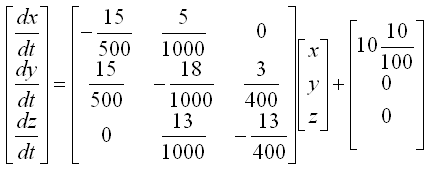DE - Modeling                                                                          Home : www.sharetechnote.com The Modeling examples in this page are :     Example : Mixing Problem   This is one of the most common problems for differential equation course. You will see the same or similar type of examples from almost any books on differential equations under the title/label of "Tank problem", "Mixing Problem" or "Compartment Problem". But I think (hope) I will be providing the most detailed / step-by-step explanation -:)   To be honest I was not so good at solving this kind of problems when I first studying the problem even though it is only simple first order differential equation. Come to think of it, the reason why I was not so good at this was because I didn't clearly understand the steps through which we generate a differential equation from the description of the problem. That is, I was not so good at the modeling process.   One example for this kind of problems is as follows : i) There is a tank which has a mixture of Water and Alcohole. (Total volume of the mixture(solution) is 1000 liter). ii) The initial concentration of Alcohol is 10 % (it means that Water is solvent and Alcohol is solute) iii) There is an outlet through which the solution is going out at the rate of 50 liter/min. iv) There is an inlet through which a solution (mixture of water and alcohol) is coming in at the rate of 50 liter/min. The concentration of the alcohol in the incoming solution is 50%. The question is what will be the concentration of the alcohol of the solution in the tank after 10 mins ?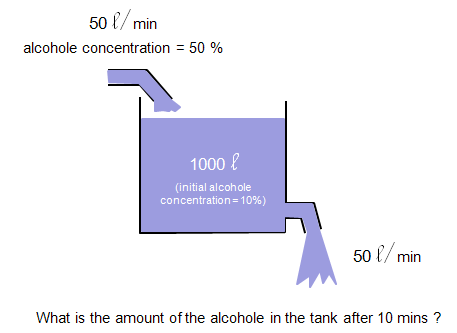The process of deducing the differential equation (modeling) is as follows. I think you have already went through a couple of examples of this kind in your Differential equation course (or you can search several tutorials of this kind in the internet) and see if the following process that I put down makes any sense to you.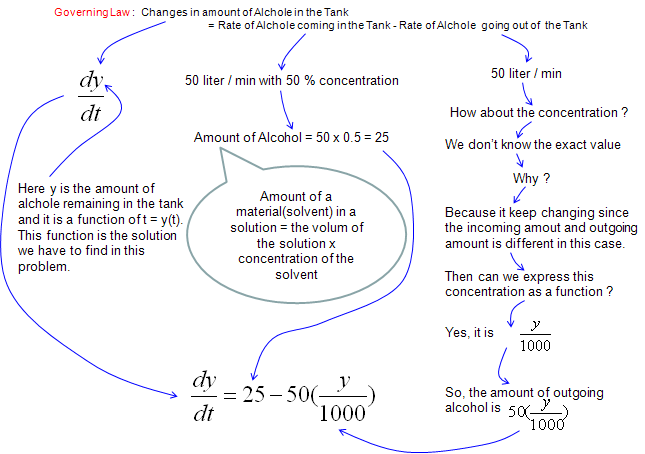Note : Don't you have any question at this point ? If you have the question saying "In the description of the problem, I see the statement 'the initial concentration of alcohol in the tank is 10%', but I don't see this condition is being used in the derivation of the equation. Does this mean that this initial condition is useless ?" We don't need the initial condition at least for modeling process (the process of deriving the differential equation), but we need the initial condition when we solve the equation to get a specific values from the solution. (In this problem, the initial condition y(0) = 1000 liter of solution x 0.1 (10 % of concentration of alcohol) = 100).     Example : Mixing Problem (Two Tank)   If you haven't read the previous example (One Tank example), please go through it first and get back to this example and try to find what is the difference and what is common. and more importantly try to find some pattern of extending the logic of thinking on modeling.   Now we have another tank (the second tank) get connected to the first tank that I used in previous example. First, just take brief look at the diagrame espectiall the direction of arrows.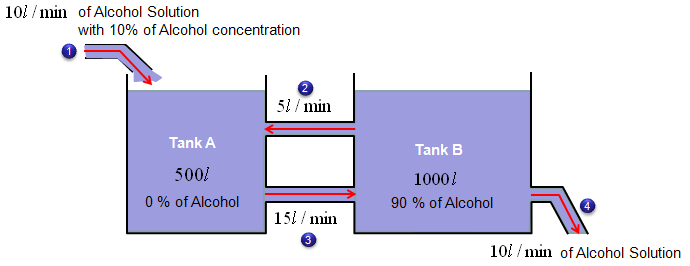i) There are two tanks which has a mixture of Water and Alcohole. (Total volume of the mixture(solution) of Tank A is 500 liter and the volume of Tank B is 1000 litter). ii) The initial concentration of Alcohol for Tank A is 0 % (it means that pure Water) iii) The initial concentration of Alcohol for Tank B is 90 % iv) There is an outlet for Tank A through which the solution is going out at the rate of 15 liter/min. v) There are two outlets for Tank B and the solution is going out at the rate of 5 liter/min through one outlet and the solution is going out at the rate of 10 liter/min through the other outlet. vi) There are two inlets for Tank A. A solution (mixture of water and alcohol) is coming in at the rate of 10 liter/min with the concentration of 10 % through one inlet and solution (mixture of water and alcohol) is coming in at the rate of 5 liter/min with the yet unknown concentration through one inlet. vii) There are one inlet for Tank B. A solution (mixture of water and alcohol) is coming in at the rate of 15 liter/min with the yet unknown concentration through the inlet.     The question is what will be the concentration of the alcohol of the solution in the tank A and Tank B after 10 mins ?   Before jumping into the modeling process, let me give you a couple of questions that can easily be overlooked but important.   Would the volume of solution for each tank change over time ? The answer is NO, the volume does not change over time. Most of this kind of problem from the text book are carefully designed so that the volum of tank does not change over time.   Why do they set the problem so that the volum of the solution in the tank does not change ? It is because the mathematical equation will simpler under that condition.   Let's summarize some of the initial conditions and define some of the variables for modeling.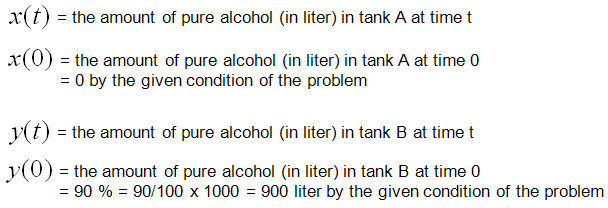Now let's look into the governing law of this problem.   The rule is as follows and you will notice that this law is exactly same as what you saw in previous example (Single Tank case). The only difference is that Tank A has one more inlet.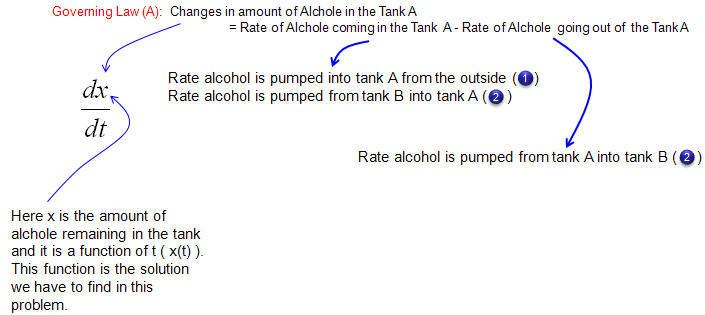Next step is to describe the amount of alcohol coming in and out of the tank A in mathmatical terms as follows. Overall the expressions are simple. The only thing you have to think a little bit is the expression for the concentration of alcohol for each tank. They are expressed as x/500 and y/1000. These can be expressed in this kind of simple manner because we set the problem so that the total amount of solution for each tank does not change over time. If the total volume of each tank changes, you cannot express it as a simple number like '500' or '1000'. You would need the functions (a function of t) describing the volume of each tank over time.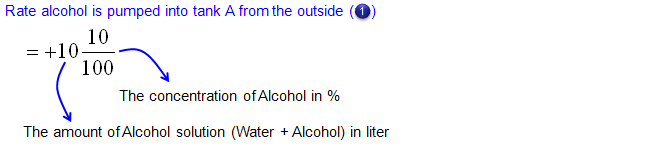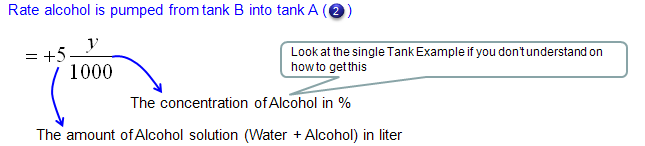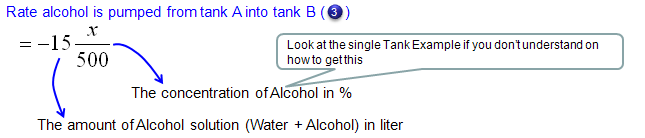Now let's look into governing law for Tank B. Overall process is as follows and you will notice that the process (logic) is exactly same as you did for Tank A.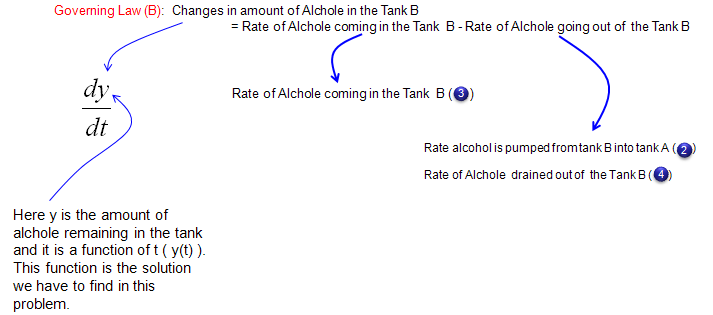Mathematical expression of each terms of governing law (governing equation) are as follows.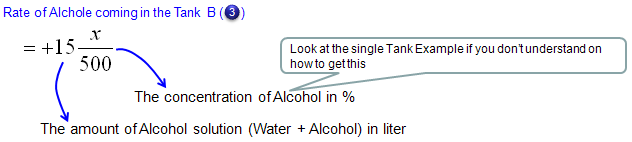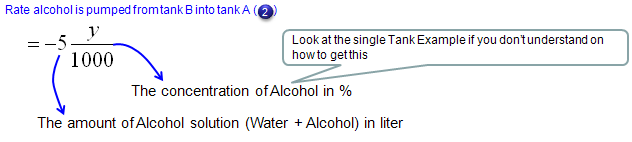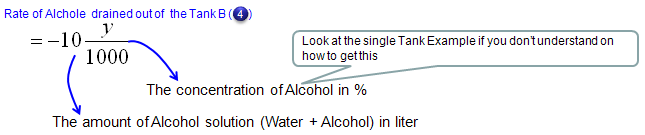Now we have each terms (components) of governing law for Tank A and Tank B. If you just combine those components according to the governing law you will get the following two differential equation.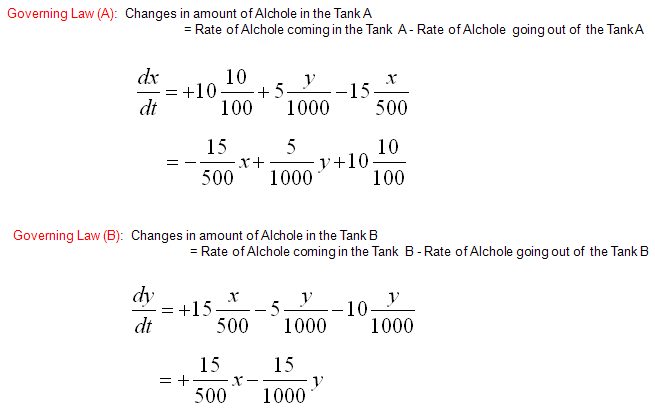Writing again the two equations into a simultaneous equation (system equation), we get the following.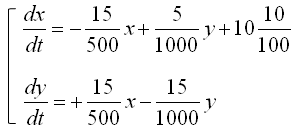This is done with modeling and you can solve the equations as it is, but some software require the system equations to be input in matrix format as follows.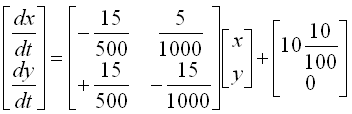Example : Mixing Problem (Three Tanks)   This is one-step further extention of the previous example. (Make it sure that you have gone through the previous two examples - single tank, two tank problems before you start this example).   Now we have another tank (the third tank) get connected to the second tank that I used in previous example. First, just take brief look at the diagrame espectiall the direction of arrows.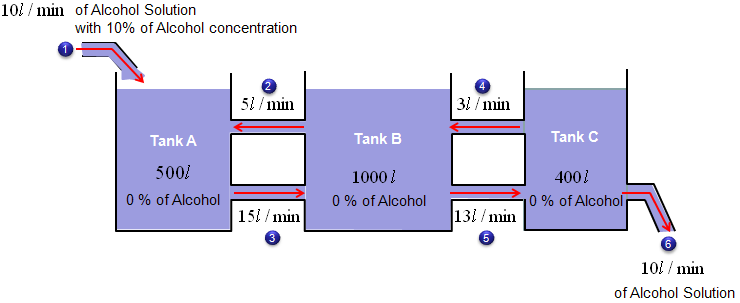i) There are three tanks which has a mixture of Water and Alcohole. (Total volume of the mixture(solution) of Tank A is 500 liter and the volume of Tank B is 1000 litter and the volume of Tank C is 400 litter). ii) The initial concentration of Alcohol for Tank A is 0 % (it means that pure Water) iii) The initial concentration of Alcohol for Tank B is 0 % (it means that pure Water) iv) The initial concentration of Alcohol for Tank C is 0 % (it means that pure Water) v) There is an outlet for Tank A through which the solution is going out at the rate of 15 liter/min. vi) There are two outlets for Tank B and the solution is going out at the rate of 5 liter/min through one outlet and the solution is going out at the rate of 13 liter/min through the other outlet. vii) There are two outlets for Tank C and the solution is going out at the rate of 3 liter/min through one outlet and the solution is going out at the rate of 10 liter/min through the other outlet. viii) There are two inlets for Tank A. A solution (mixture of water and alcohol) is coming in at the rate of 10 liter/min with the concentration of 10 % through one inlet and solution (mixture of water and alcohol) is coming in at the rate of 5 liter/min with the yet unknown concentration through one inlet. ix) There are two inlet for Tank B. A solution (mixture of water and alcohol) is coming in at the rate of 15 liter/min with the yet unknown concentration through the inlet and solution (mixture of water and alcohol) is coming in at the rate of 3 liter/min with the yet unknown concentration through one inlet..   x) There is one inlet for Tank C. A solution (mixture of water and alcohol) is coming in at the rate of 13 liter/min with the yet unknown concentration through the inlet   The question is what will be the concentration of the alcohol of the solution in the tank A,Tank B and Tank C after 10 mins ?   Before jumping into the modeling process, let me give you a couple of questions that can easily be overlooked but important.   Would the volume of solution for each tank change over time ? The answer is NO, the volume does not change over time. Most of this kind of problem from the text book are carefully designed so that the volum of tank does not change over time.   Why do they set the problem so that the volum of the solution in the tank does not change ? It is because the mathematical equation will simpler under that condition.   Let's summarize some of the initial conditions and define some of the variables for modeling.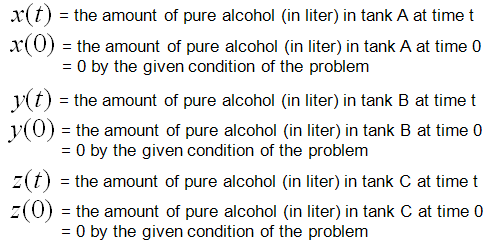Now let's look into the governing law of this problem.   The rule is as follows and you will notice that this law is exactly same as what you saw in previous example (Two Tank case).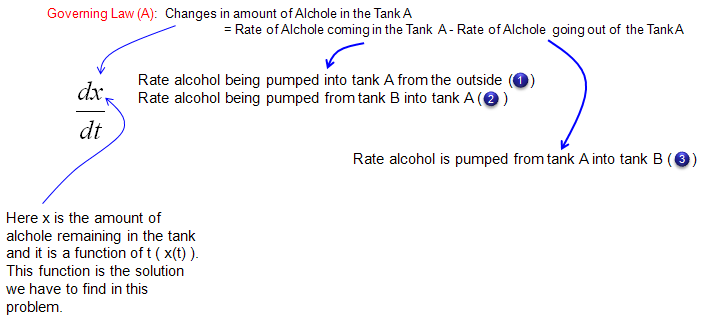Next step is to describe the amount of alcohol coming in and out of the tank A in mathmatical terms as follows. Overall the expressions are simple. The only thing you have to think a little bit is the expression for the concentration of alcohol for each tank. They are expressed as x/500 and y/1000. These can be expressed in this kind of simple manner because we set the problem so that the total amount of solution for each tank does not change over time. If the total volume of each tank changes, you cannot express it as a simple number like '500' or '1000'. You would need the functions (a function of t) describing the volume of each tank over time.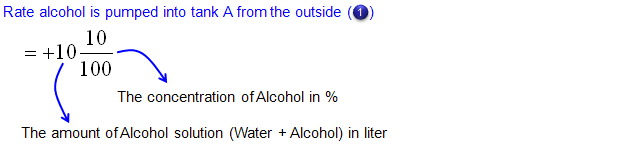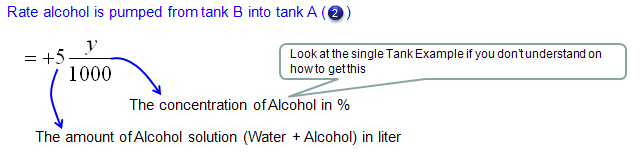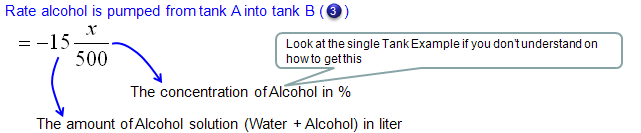Now let's look into governing law for Tank B. Overall process is as follows and you will notice that the process (logic) is exactly same as you did for Tank A.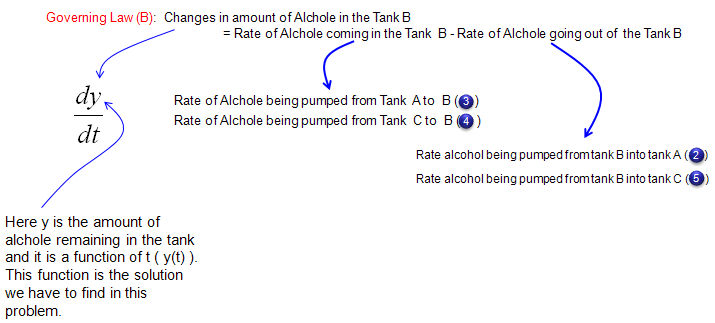Mathematical expression of each terms of governing law (governing equation) are as follows.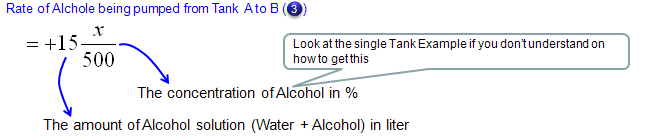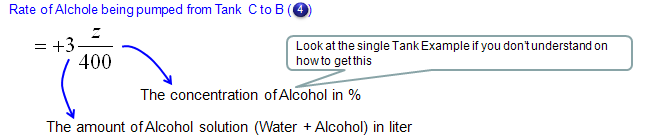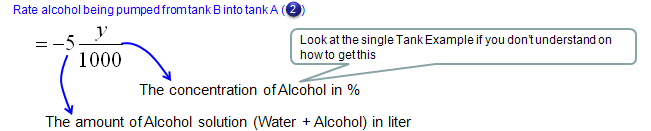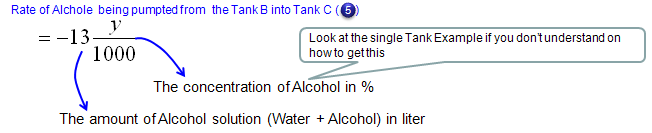Now let's look into governing law for Tank C. Overall process is as follows and you will notice that the process (logic) is exactly same as you did for Tank A.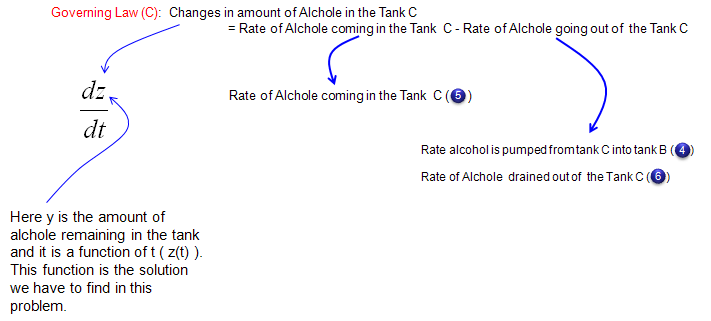Mathematical expression of each terms of governing law (governing equation) are as follows.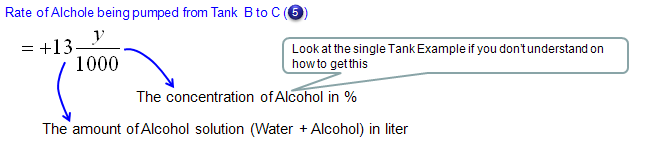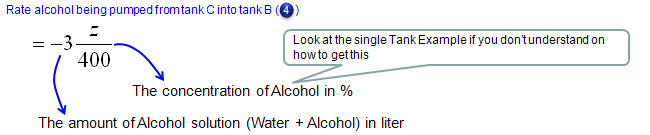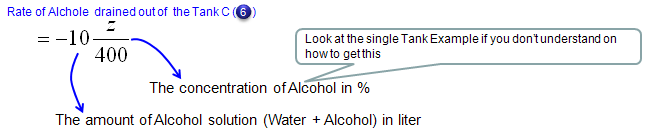Now we have each terms (components) of governing law for Tank A,Tank B and Tank C. If you just combine those components according to the governing law you will get the following three differential equation.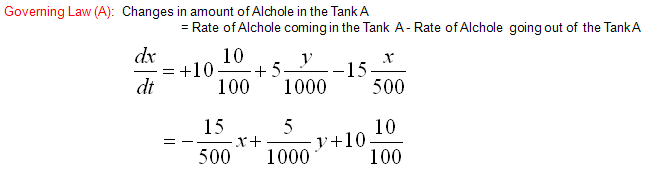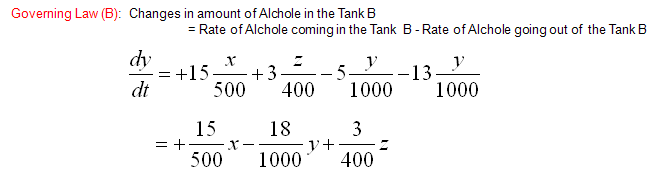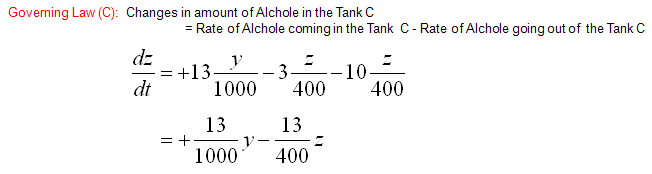Writing again the three equations into a simultaneous equation (system equation), we get the following.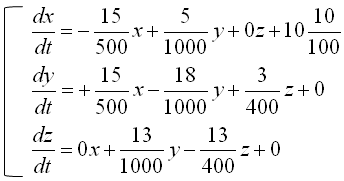This is done with modeling and you can solve the equations as it is, but some software require the system equations to be input in matrix format as follows.